﻿ End Behavior of Polynomials

# END BEHAVIOR OF POLYNOMIALS

• PRACTICE (online exercises and printable worksheets)

## End Behavior of General Functions

Roughly, the ‘end behavior’ of a function refers to what happens to its graph as you travel ...

• ... farther and farther to the right;
this is called right-hand end behavior
• ... farther and farther to the left;
this is called left-hand end behavior

‘Farther and farther to the right’ means you let $\,x\,$ go to infinity, which is written as ‘$\,x\rightarrow\infty\,$’.
‘Farther and farther to the left’ means you let $\,x\,$ go to negative infinity, which is written as ‘$\,x\rightarrow-\infty\,$’.

End behavior is studied to understand what happens to the outputs from a function (the $y$-values of points on the graph)
as you move farther and farther away from the origin.
For example, you might want to know:

• Are the outputs getting bigger and bigger, without bound?
• Are the outputs getting closer to zero?
• Are the outputs approaching some nonzero number?

In general, functions can have a variety of end behaviors, as shown in the table below.
Only right-hand end behaviors are shown here.

increasing without bound
as you move to the right:
as $x\rightarrow\infty\,$,
$\,y\rightarrow\infty$

‘as $\,x\,$ goes to infinity,
$\,y\,$ goes to infinity’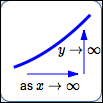as in $\,y = x^2\,$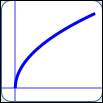as in $\,y = \sqrt{x}\,$as in $\,y = x\,$
decreasing without bound
as you move to the right:
as $x\rightarrow\infty\,$,
$\,y\rightarrow-\infty$

‘as $\,x\,$ goes to infinity,
$\,y\,$ goes to negative infinity’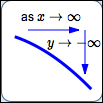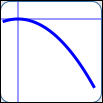as in $\,y = -x^2\,$as in $\,y = -\sqrt{x}\,$as in $\,y = -x\,$
approaching a particular real number (like $\,3\,$)
as you move to the right:
as $x\rightarrow\infty\,$,
$\,y\rightarrow 3$

‘as $\,x\,$ goes to infinity,
$\,y\,$ approaches $\,3\,$’as in $\displaystyle\,y = 3 + \frac 1x$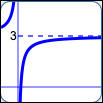as in $\displaystyle\,y = 3 - \frac 1x\,$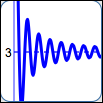as in $\displaystyle\,y = 3 + \frac{\sin x}{x}\,$

## End Behavior of Polynomials

Polynomials can't exhibit the full variety of end behavior illustrated above.
As $\,x\,$ gets large, $\,y\,$ can only do two things: go to infinity, or go to negative infinity.
The best news is that we only need one term of the polynomial to figure out what the end behavior is!

KEY IDEA:
For large values of $\,x\,$, a polynomial ‘behaves like’ its highest power term.

For example, suppose you're investigating the end behavior of $\,P(x) = x^3 - 3x^2 + 5x - 10\,$.
Notice that:

• the degree of $\,P\,$ is $\,3\,$
• the leading coefficient is $\,1\,$
• the highest power term is $\,x^3\,$
To investigate the end behavior of $\,P\,$, you can instead work with the much simpler polynomial $\,Q(x) = x^3\,$.

Why?
A renaming of $\,P(x)\,$ does the trick, where we factor out the highest power of $\,x\,$: $$P(x) = x^3 - 3x^2 + 5x - 10 = x^3\left(1 - \frac 3x + \frac 5{x^2} - \frac{10}{x^3}\right)$$ From this new name, it is clear that as $\,x\,$ gets big, all the terms go to zero except the highest power term.
Roughly, the highest power term ‘washes out’ all the other terms when $\,x\,$ is big.

Even when $\,x\,$ is as small as $\,x = 50\,$, let's investigate each of the terms in $\,P(x)\,$:

• $x^3 = 50^3 = 125000$
• $-3x^2 = -3\cdot 50^2 = -7500$
• $5x = 5\cdot 50 = 250$
• $-10$
• $P(50) = 125000 - 7500 + 250 - 10$
How significant are those other terms compared to the first? Not!
The highest power term determines the behavior of the output when the input is large.

KEY IDEA:
The leading coefficient and degree of a polynomial determine its end behavior.

All possible end behaviors of polynomials are summarized in the following table.

END BEHAVIORS OF POLYNOMIALS

Consider a polynomial with degree $\,n\,$ and leading coefficient $\,a\,$.
Thus, the highest power term is $ax^n$.
degree
($n$)
($a$)
example end behavior
$n$ even:

$n = 2,\, 4,\, 6,\, \ldots$
$a > 0$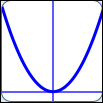as in $\,y = 3x^2\,$:   $a = 3\,$,   $n = 2$
 right-hand: as $\,x\rightarrow\infty\,$, $y\rightarrow\infty$ left-hand: as $\,x\rightarrow-\infty\,$, $y\rightarrow\infty$
$a < 0$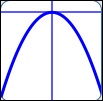as in $\,y = -3x^2\,$:   $a = -3\,$,   $n = 2$
 right-hand: as $\,x\rightarrow\infty\,$, $y\rightarrow-\infty$ left-hand: as $\,x\rightarrow-\infty\,$, $y\rightarrow-\infty$
$n$ odd:

$n = 1,\, 3,\, 5,\, \ldots$
$a > 0$as in $\,y = 2x^3\,$:   $a = 2\,$,   $n = 3$
 right-hand: as $\,x\rightarrow\infty\,$, $y\rightarrow\infty$ left-hand: as $\,x\rightarrow-\infty\,$, $y\rightarrow-\infty$
$a < 0$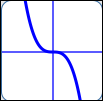as in $\,y = -2x^3\,$:   $a = -2\,$,   $n = 3$
 right-hand: as $\,x\rightarrow\infty\,$, $y\rightarrow-\infty$ left-hand: as $\,x\rightarrow-\infty\,$, $y\rightarrow\infty$

## Example

Let $\,P(x) = 2x^3 - 5x^7 + 6x - 5\,$.
Determine the end behavior of $\,P\,$.

SOLUTION:

The highest order term is $\,-5x^7\,$.
For large values of $\,x\,$, $\,P(x)\approx -5x^7\,$.

Think of substituting a large positive number into $\,-5x^7\,$.
The sign of the output is:   $(-)(+)^7 = (-)$
The output is a large negative number, so:
as $\,x\rightarrow\infty,\ \ P(x)\rightarrow -\infty$

Think of substituting a large negative number into $\,-5x^7\,$.
The sign of the output is:   $(-)(-)^7 = (-)(-) = (+)$
The output is a large positive number, so:
as $\,x\rightarrow-\infty,\ \ P(x)\rightarrow \infty$

## Making End Behavior Precise

Mathematical sentences like ‘as $\,x\rightarrow\infty\,$, $\,y\rightarrow\infty\,$’ can be made precise in a Calculus course.
For those of you who are curious right now:
 $\text{as } x\rightarrow\infty,\ y\rightarrow\infty$ is equivalent to for every $\,N > 0\,$ there exists $\,M > 0\,$ such that whenever $\,x > M\,$, $y > N$

Master the ideas from this section